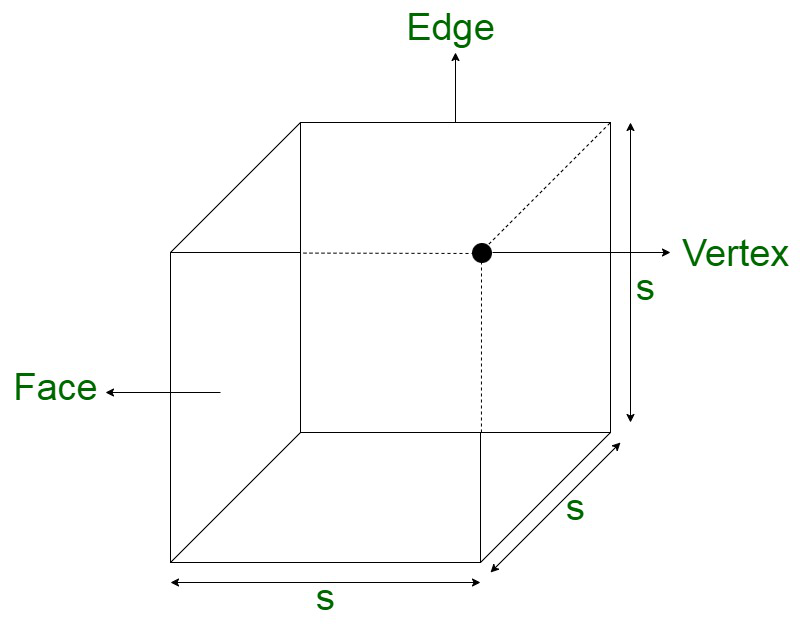# What will happen to the volume of a cube if its edge is reduced to one fourth?

• Last Updated : 11 Nov, 2021

Cube is a three-dimensional solid object. It is also referred to as a regular hexahedron and is one of the five platonic solids. All the edges share at least a common edge with each other. The structure of a cube can be defined in terms of the fact that each of the faces is being connected to four vertices and four edges, vertex connected with three edges and three faces, and edges are in touch with two faces and two vertices.Properties of Cube

• All the square faces are equal.
• All the sides are equal in length.
• Comprises of eight vertices and twelve edges.
• Length = Breadth = Height

### Volume of Cube

The total volume occupied by the cube is referred to as its volume. Since a cube has all similar faces, therefore, the length of edges is also equivalent. Therefore, the length, width, and height of the cube are of equal length. The volume of the cube is defined in terms of the cubic units that are occupied by the geometrical figure completely.

The volume of the cube can be computed using the length of the edges of the cube. Let us assume the side of the cube to be a. Let us also assume V to be the volume of the cube. So, the volume of the cube formula is:

Volume of a Cube = Length × Width × Height

Since, all the sides are equal in cube.

Volume = a × a × a

Volume = a3

### What will happen to the volume of a cube if its edge is reduced to one-fourth?

The formula used in solving this problem is,

Volume of the Cube,  V = a3

Let us assume the side of the cube to be a.

Let us assume the volume of the cube to be ‘V’.

Now, we know,

The edge of the cube is reduced to one fourth

This implies that,

a’ = a/4

Volume of the cube, V’ =On simplifying, we get,The volume of the Cube when the edge is reduced to one fourth istimes the original volume.

### Sample Questions

Question 1. How many times the volume of the cube is reduced if its edge is reduced by?

Solution:

Here we have to find how much volume will be reduced if the edge of a cube is reduced byAssume a is the side of the cube

If the side of cube is reduced bythen,

a ==As we know that,

Volume of cube V =  a × a × a = a3

Volume of the reduced edge cube V’ =Volume of the reduced edge cube V’ =Volume of the reduced edge cube V’ =Volume of the reduced edge cube V’ =Volume of the reduced edge cube V’ =Therefore,

We can see that if we reduce the edge of the cube bythe volume of the cube is reduced bytimes.

Question 2. By how much the volume of a cube will increase if its edge is increased by four times?

Solution:

Here we have to find the change in the volume of the cube when its edge is increased by four times.

Assume a is the side of the cube

If the side of the cube is four times

a = 4 × a = 4a

As we know that,

Volume of cube V =  a × a × a = a3

Volume of cube with four times side V’ =  4a × 4a × 4a

Volume of cube with four times side V’ =  64a3

Volume of cube with four times side V’ =  64 × V

Therefore,

We can see that if we increase the edge of the cube by four times its volume is increased by 64 times.

Question 3. By how much the volume of a cube will decrease if we reduce the edge of the cube by halved?

Solution:

Here we have to find how much volume will be reduced if the edge of a cube is reduced by halve

Assume a is the side of the cube

If the side of the cube is reduced by halve then,

a =As we know that,

Volume of cube V =  a × a × a = a3

Volume of the reduced edge cube V’ =Volume of the reduced edge cube V’ =Volume of the reduced edge cube V’ =Volume of the reduced edge cube V’ =Volume of the reduced edge cube V’ =Therefore,

We can see that if we reduce the edge of the cube by half the volume of the cube is reduced bytimes.

Question 4. If the volume of the cube is 1728 cm3. Then find the side of the cube.

Solution:

Here we have to find the length of the side of the cube,

Assume the side of the cube is ‘a’

As we know that,

Volume of cube  =  a × a × a = a3

In the question, we are given the volume of the cube as 1728 cm3

1728 = a × a × a = a3

1728 = a3

a =a = 12 cm

Therefore,

Side of the cube with volume 1728 cm2 is 12 cm.

Question 5. A cubical box has to be filled with sand of side 15 cm. Find the volume of the sand that will be required to fill the cubical box?

Solution:

Here we have to find the sand that can be filled in the cubical box

We are given that the side of the room is 15 cm

As we know that

Volume of the cube = a × a × a

Volume of the cube = a3

Volume of the cube = 153

Volume of the cube = 3375 m3

Therefore,

3375 m3 sand can be filled in a cubical box of size 15 cm.

My Personal Notes arrow_drop_up